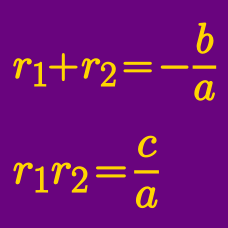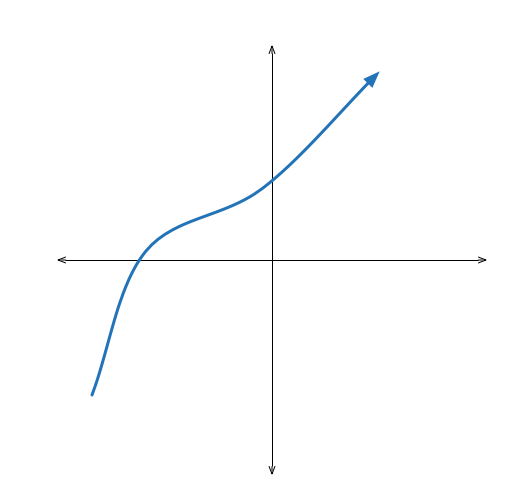Algebra

# Vieta's Formula: Level 3 Challenges

Jason and Thompson were solving the quadratic equation $x^{2} + bx + c = 0$.

Jason wrote down the wrong value of $b,$ and found the roots to be 6 and 1. Thompson wrote down the wrong value of $c,$ and found the roots to be $-4$ and $-1.$

What are the actual roots of the equation?

The non-zero roots of

$ax^2+bx+c=0$

are $r$ and $s.$ What are the roots of

$cx^2+bx+a = 0?$The roots of the polynomial equation $x^3 + 2 x^2 + 3 x + 4 = 0$ are $\alpha, \beta,$ and $\gamma$. What is the value of

$\frac{ \alpha + \beta} { \gamma} + \frac{ \beta + \gamma} { \alpha } + \frac{ \gamma + \alpha } { \beta } ?$

If $\alpha$, $\beta$, and $\gamma$ are the roots of $x^3-x-1=0$, compute:

$\frac{1-\alpha}{1+\alpha}+\frac{1-\beta}{1+\beta}+\frac{1-\gamma}{1+\gamma}$

$\large (x-7)(x-3)(x+5)(x+1)=1680$

Find the sum of all $x$ that satisfy the equation above.

×